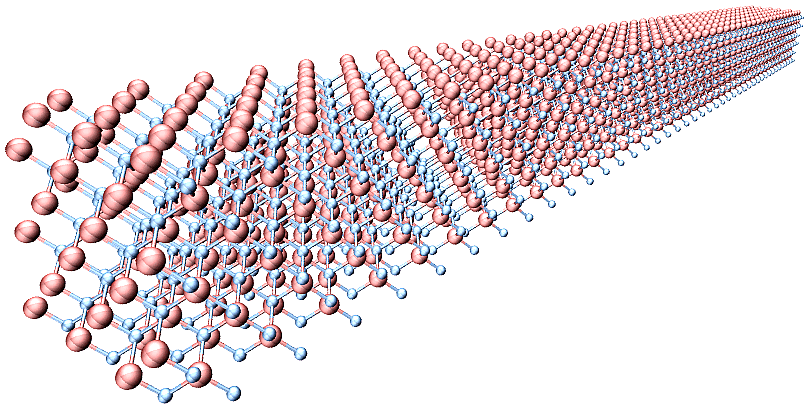Japanese

# Tsuneyuki Research Group

## Research

### "First-principles calculation of lattice thermal conductivity"In solids, thermal energy is mainly carried by electrons and phonons, latter of which becomes dominant in insulators and semiconductors. The magnitude of the lattice thermal conductivity $$\kappa_{L}$$ varies significantly for different materials and is closely related to the lattice anharmonicity. For example, the diamond shows very high lattice thermal conductivity ($$\kappa_{L} > 1000$$ W/mK) because its lattice vibration is quite harmonic. Conversely, the $$\kappa_{L}$$ value of an ionic compound PbTe is very small ($$\kappa_{L} \sim 1$$ W/mK), which can be attributed to the strong phonon scattering resulting from the large lattice anharmonicity. Developing a non-empirical method to calculate lattice thermal conductivity is desired not only for gaining a robust understanding of thermal transport phenomena in solids but also for optimizing the figure-of-merit of thermoelectric materials.

Our research group has been developing a versatile method to compute lattice thermal conductivity of solids. In our approach, we first express the potential energy surface of the interacting atomic system as a Taylor expansion with respect to the atomic displacements from equilibrium positions $$\{u_{i}\}$$. The coupling coefficients are harmonic and anharmonic interatomic force constants (IFCs), which are determined by first-principles calculations based on density-functional theory (DFT). The estimated IFCs are then employed to calculate the lattice thermal conductivity either by conducting a (non-equilibrium) molecular dynamics simulation or by solving the Boltzmann transport equation. We applied the method to Si and a thermoelectric material Mg2Si and obtained reasonable $$\kappa_{L}$$ values that were comparable with experimental results . The computational code developed by our group is now distributed as an open-source software , which can be used with any DFT packages, such as VASP and Quantum-ESPRESSO, to predict the lattice thermal conductivity.

 T. Tadano, Y. Gohda, and S. Tsuneyuki, J. Phys.: Condens. Matter 26, 225402 (2014).